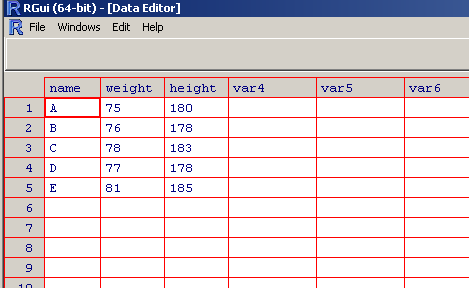Install "foreach" library:  install.packages("foreach", repos="http://R-Forge.R-project.org")

Then load it:  library(foreach) For documentation, you may see this file: https://cran.r-project.org/web/packages/foreach/vignettes/foreach.pdf

A few examples from the above file:

x <- foreach(i=1:3) %do% sqrt(i)

The above will compute the squareroots of 1, 2 and 3: 1, 1.414214 and 1.732051

The code x <- foreach(a=x, b=rep(10, 3)) %do% (a + b) will add 10 for each components of x: 11, 11.414214 and 11.732051

Noe install: install.packages("resample") and load it library(resample)

We can combine vectors into a data frame, see the following code and output:

It is not pleasant to edit data this way, so we should use R GUI to do this:

group <- edit(group)

After you hit return key, a data entry form will appear. It will looks like this:Now edit by adding a row and a column as shown by the following picture:Once you close this window, the change will be automatically saved. You can verify.  In addition, you can use "fix" to open the data window. See the following code and output.